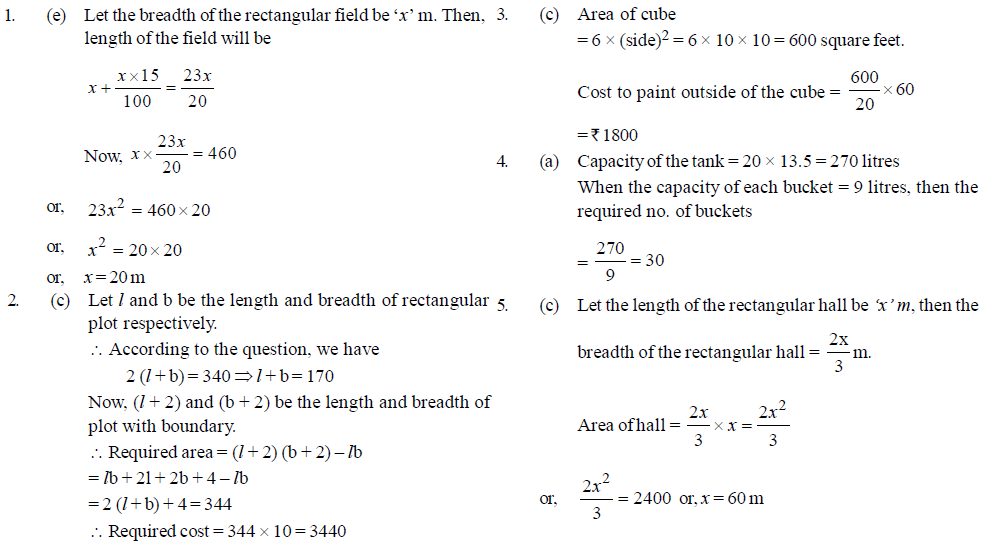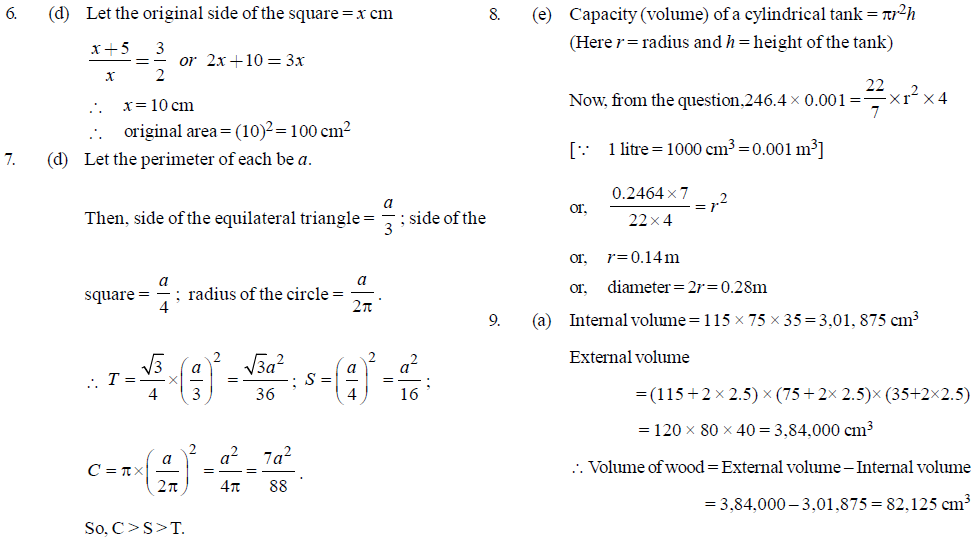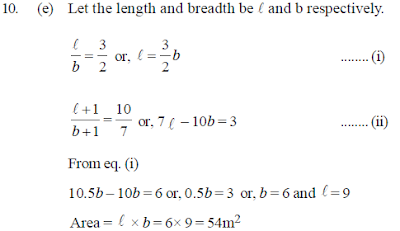## Mensuration Practice Quiz – Set 1

Mensuration Practice Quiz – Set 1
1. The area of rectangular field is 460 square metres. If the length is 15 per cent more than the breadth, what is the breadth of the rectangular field?
(a) 15 metres
(b) 26 metres
(c) 34.5 metres
(d) Cannot be determined
(e) None of these
2. What will be the cost of gardening 1-metre – broad boundary around a rectangular plot having perimeter of 340 metres at the rate of Rs.10 per square metre?
(a) Rs.3400
(b) Rs.1700
(c) Rs.3440
(d) Cannot be determined
(e) None of these
3. The cost of paint is Rs.60 per kilogram. A kilogram paint covers 20 square feet. How much will it cost to paint the outside of a cube having each side 10 feet?
(a) Rs.3000
(b) Rs.900
(c) Rs.1800
(d) Rs.360
(e) None of these
4. 20 buckets of water fill a tank when the capacity of each bucket is 13.5 litres. How many buckets will be required to fill the same tank if the capacity of each bucket is 9 litres?
(a) 30
(b) 32
(c) 60
(e) None of these
5. The breadth of a rectangular hall is two-thirds of its length. If the area of the hall is 2400 sq metres, what is the length in metres?
(a) 120
(b) 80
(c) 60
(d) 40
(e) None of these
6. If a pair of opposite sides of a square is increased by 5 cm each, then the ratio of the sides of the new figure is 3 : 2. What is the original area of the square?
(a) 125 cm^2
(b) 225 cm^2
(c) 81 cm^2
(d) 100 cm^2
(e) None of these
7. An equilateral triangle, a square and a circle have equal perimeters. If T denotes the area of the triangle, S, the area of the square and C, the area of the circle, then :
(a) S > T > C
(b) T > C > S
(c) T > S > C
(d) C > S > T
(e) None of these
8. The capacity of a cylindrical tank is 246.4 litres. If the height is 4 metres, what is the diameter of the base?
(a) 1.4 metres
(b) 2.8 metres
(c) 28 metres
(d) 14 metres
(e) None of these
9. The internal measurements of a box with lid are 115 × 75 × 35 cm^3 and the wood of which it is made is 2.5 cm thick. Find the volume of wood.
(a) 82,125 cm^3
(b) 70,054 cm^3
(c) 78,514 cm^3
(d) None of these
(e) None of these
10. The length and the breadth of a rectangle are in the ratio of 3 : 2 respectively. If the sides of the rectangle are extended on each side by 1 metre, the ratio of length to breadth becomes 10 : 7. Find the area of the original rectangle in square metres.
(a) 56
(b) 50
(c) 80